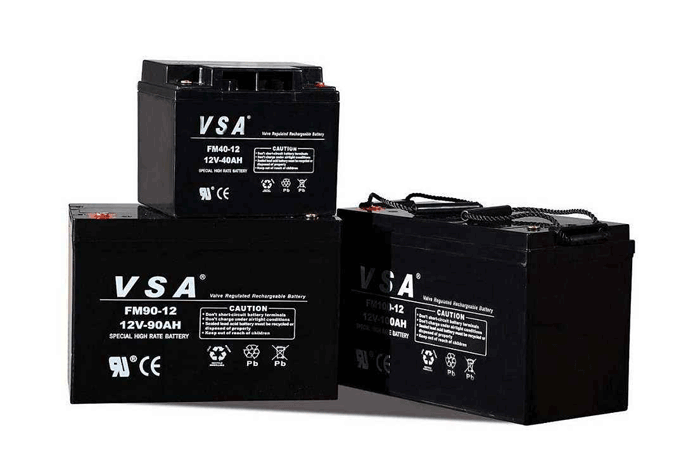Technical articles

# 关于UPS蓄电池容量计算与蓄电池配置介绍

UPS蓄电池容量计算方法很多，各行各业都有相应的计算方法选择的侧重点，下面收集UPS行业中常用的几种计算方法，注意到现有部份行业中UPS系统的负荷当电力出现问题时，负荷会分时段变化，此类系统中蓄电池容量的计算与选择也是众说纷纭，在此提供计算方式供大家讨论。1、恒功率法(查表法)计算UPS蓄电池容量

P负荷=[P(VA)×Pf]/η

①P负荷=[P(VA)×Pf]/η
②Pnc=P负荷/(N×n)

2、估算法计算UPS蓄电池容量

I电池=W电池/(U电池×T)=P电池/U电池
C10=I电池/KCh

Imax=[P(VA)×Pf]/(η×Umin)
C10=I/KC

3、电源法计算UPS蓄电池容量

I=[P(VA)×Pf]/µU
Q≥KIT/H(1+A(t-25))80KVA UPS后备时间30min，如何计算电池容量？

80KVA UPS直流终止电压为300V及U临界=300V，直流电压为348V电池组选用29只12V电池，故N=29，n=6，U终压=1.75V，UPS的功率因子Pf=0.8，逆变器转换效率η=0.95。

1、恒功率法计算UPS蓄电池容量实例
P(W)=[P(VA)×Pf]/η=[80×1000×0.8]/0.95=67368.4(W)
Pnc=P(W)/(N*n)=67368.4/(29×6)=387.2(W)

2、估算法计算UPS蓄电池容量实例
Imax=[P(VA)×Pf]/(η×Umin)=(80×1000×0.8)/(0.95×300)=224.6(A)

C10=I/KCh=224.6A/0.98=229(Ah)

3、电源法计算UPS蓄电池容量实例
Imax=[P(VA)×Pf]/(η×U临界)=(80×1000×0.8)/(0.95×300)=224.6(A)
Q≥KIT/≥1.25×224.6×0.5/≥438ah
30min电池的容量换算系数取0.4，(引用该电池不同放电时率不同放电终止电压下，电池的容量换算表)，温度为25℃。

• 地址：山东省济南市天桥区粟山路10号

• 电话：053181767169

•15668249909